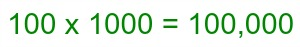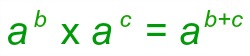# 100 x 1000: How-To & Steps

Instructor: Laura Pennington

Laura received her Master's degree in Pure Mathematics from Michigan State University, and her Bachelor's degree in Mathematics from Grand Valley State University. She has 20 years of experience teaching collegiate mathematics at various institutions.

In this lesson, we will look at the steps to solve the multiplication problem 100 x 1000, explain why the steps work, and learn how to extend the process to other problems of this type.

## Steps to Solve

Let's look at how to multiply 100 x 1000. The process to solve this problem is actually quite simple; to multiply 100 times 1000, take the following steps:

1. Count the number of zeros in 100.
2. Count the number of zeros in 1000.
3. Add up the number of zeros you counted in step 1 and 2.
4. Write the number 1, and then follow it with the number of zeros you found in step 3.

Notice that each step is very easy to perform. That's the great thing about breaking a problem down into steps - if you just take it one step at a time, it's really quite simple!

Let's take the problem through the steps to find our answer. The first step is to count the number of zeros in 100 - there are 2 zeros in 100. The next step is to count the number of zeros in 1000 - there are 3 zeros in 1000. Now, add up the number of zeros we found in steps 1 and 2:

2 + 3 = 5

We have 5 zeros total. The last step is to write the number 1 and follow it with the number of zeros found, or 5 zeros:

100000

## Solution

When we multiply 100 by 1000, we get 100,000.## Solving Method Explained Using Exponents.

You're probably glad to know how to solve the problem, but you may be wondering why this process works. Well, don't worry! We're going to explain it using exponents.

To understand this process, we need to be familiar with a couple of facts. The first is writing 100 and 1000 as powers of 10. Observe the following:

100 = 10 x 10 = 10 2

1000 = 10 x 10 x 10 = 10 3

We know that 100 = 10 2 and that 1000 = 10 3. Notice that the exponents are equal to the number of zeros in the number. Basically, to write these numbers as powers of 10, count the number of zeros in the number, then raise 10 to that power. This corresponds to the first and second steps of the solving process, and we find the following:

100 x 1000 = 10 2 x 10 3

The next fact that we need to be familiar with is the multiplication rule of exponents. This rule states thatWe know that a b x a c = a b+c. We can apply this to multiplying 10 2 x 10 3. This corresponds to the third step of the solving process, and we find the following:

100 x 1000 = 10 2 x 10 3 = 10 2+3 = 10 5

The last step in the solving process is calculating 10 5, which is 100000. Altogether, we find that:

100 x 1000 = 10 2 x 10 3 = 10 2+3 = 10 5 = 100000

To unlock this lesson you must be a Study.com Member.

### Register to view this lesson

Are you a student or a teacher?